# Lattice-ordered group

(diff) ← Older revision | Latest revision (diff) | Newer revision → (diff)-group

A groupon the set of elements of which a partial-order relation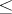is defined possessing the properties: 1)is a lattice relative to, i.e. for any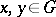there are elements,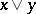such that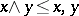and; for any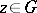,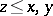implies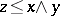, and for anyandone has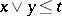; and 2) for any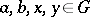the inequality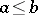implies. Similarly, a lattice-ordered group can be defined as an algebraic system of signature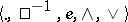that satisfies the axioms: 3)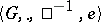is a group; 4)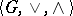is a lattice; and 5)and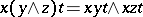for any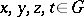.

The lattice of elements of a lattice-ordered group is distributive (cf. Distributive lattice). The absolute value (respectively, the positive and the negative part) of an elementis the element(respectively,and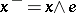). In lattice-ordered groups, the following relations hold: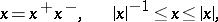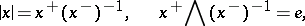Two elementsandare called orthogonal if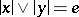. Orthogonal elements commute.

A subsetof an-groupis called an-subgroup ifis a subgroup and a sublattice in; an-subgroupis called an-ideal ofif it is normal and convex in. The set of-subgroups of a lattice-ordered group forms a sublattice of the lattice of all its subgroups. The lattice of-ideals of a lattice-ordered group is distributive. An-homomorphism of an-groupinto an-groupis a homomorphismof the groupinto the groupsuch that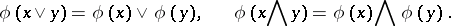The kernels of-homomorphisms are precisely the-ideals of-groups. Ifis an-group and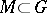, then the set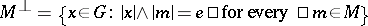is a convex-subgroup in(cf. Convex subgroup).

The group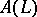of one-to-one order-preserving mappings of a totally ordered setonto itself is an-group (if forone assumes thatif and only if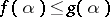for all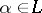). Every-group is-isomorphic to an-subgroup of the lattice-ordered groupfor a suitable set.

The class of all lattice-ordered groups is a variety of signature(cf. Variety of groups). Its most important subvariety is the class of lattice-ordered groups that can be approximated by totally ordered groups (the class of representable-groups, cf. also Totally ordered group).

How to Cite This Entry:
Lattice-ordered group. Encyclopedia of Mathematics. URL: http://encyclopediaofmath.org/index.php?title=Lattice-ordered_group&oldid=15070
This article was adapted from an original article by V.M. Kopytov (originator), which appeared in Encyclopedia of Mathematics - ISBN 1402006098. See original article